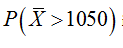# A sample of n=16 observations is drawn from a normal population with µ=1,000 and σ = 200.  Find the following.P(> 1,050)P(< 960)P(> 1,100)Please do not solve using Microsoft Excel because my professor does not teach using excel and I would like to see the steps involved in solving the equation

Question
1. A sample of n=16 observations is drawn from a normal population with µ=1,000 and σ = 200.  Find the following.
1. P(> 1,050)
2. P(< 960)
3. P(> 1,100)

Please do not solve using Microsoft Excel because my professor does not teach using excel and I would like to see the steps involved in solving the equation

check_circleExpert Solution
Step 1

From the given data

The populations mean

The standard deviation

The sample size

Step 2

Here to find the value ofis as following manner

Here

Step 3

Then plugging th...

### Want to see the full answer?

See Solution

#### Want to see this answer and more?

Solutions are written by subject experts who are available 24/7. Questions are typically answered within 1 hour*

See Solution
*Response times may vary by subject and question
Tagged in

### Statistics